# WEEKNUM in ExcelArticle byExcelMojo Team## What Is WEEKNUM Function In Excel?

The WEEKNUM function in Excel is an inbuilt Date & Time function that determines the week number of the specified date. And the function uses week numbering systems, System 1 or System 2, to decide the first week of the year required for calculating the week number.

Users can utilize the WEEKNUM function while deciding task schedules and project timelines.

For example, the table below contains a list of dates, and column C shows the day to consider when the week begins for each corresponding date value.

And suppose the requirement is to calculate the week number of each date in column A, considering the day the week begins in each case, and display the results in column D.

Then, we can get the required data using WEEKNUM in Excel column D. We shall assume the WEEKNUM function follows System 1 while determining the required week numbers. The next section will explain the week-numbering systems in detail.

In the above function WEEKNUM example, all the WEEKNUM formulas take two arguments as input, except for the formula in cell D2. The reason is that 1 is the default value of the second argument. And so, we can ignore it.

The WEEKNUM() computes the week number of the supplied date based on the day the week begins. For example, in the case of the date 1/10/2022, the week begins on Sunday. Thus, considering the week containing January 1st as week 1, and a week spans from Sunday to Saturday, the specified date falls in week three. And thus, the WEEKNUM function returns the week number as 3.

###### Key Takeaways
• The WEEKNUM function computes the week number of the given date. It follows System 1 or 2 to decide the year’s first week required while evaluating the week number.
• Users can use the WEEKNUM() to schedule weekly team activities, as the function helps calculate the week where the specified date falls. In addition, the function also helps determine the week number of a given date within the respective month.
• The WEEKNUM() accepts one mandatory argument, serial_number, and one optional argument, return_type, as input.
• Using the WEEKNUM() with other Excel functions such as SUMIFS, DATE, MONTH, and YEAR yields excellent results.

### WEEKNUM() Excel Formula

The syntax of WEEKNUM in Excel is

where,

• serial_number: The date we need to determine the week number for using WEEKNUM in Excel.
• return_type: The value that indicates the day the week begins.

While the first argument in the WEEKNUM function is mandatory, the second argument is optional.

The table below contains the values we can supply as the second argument and their interpretation.

Further, adhere to the points below to avoid the WEEKNUM function not working.

• Ensure to provide the date value as the first argument to the WEEKNUM function using the DATE(), cell reference to a date value, or as a formula returning a valid date value.
• If we ignore the second argument, the WEEKNUM function will take its default value as 1.
• Suppose the serial_number argument is out of the current date base value range, or we supply an invalid return_type argument value. Then, the WEEKNUM function will throw the #NUM! error.
• Suppose the supplied serial_number argument value is non-numeric. Then the function WEEKNUM in Excel returns the #VALUE! error.

### How To Use WEEKNUM Excel Function?

The steps to use the WEEKNUM Excel function are as follows:

1. First, confirm if the source data contains the required and valid date value.

2. Then, select the required target cell and enter the WEEKNUM function.

3. Finally, press Enter to view the week number of the given date.

The following example will explain the above steps in detail to avoid the scenarios of the WEEKNUM function not working.

The table below contains a list of requirements in column A. Some cells in column B show the dates specified in the descriptions. The table also provides the system and the first day of the week details in columns C and D.

And suppose the requirement is to calculate the week number of each specified date in the description and display the output in column E. Then, applying the WEEKNUM function in the target cells will fetch the required data.

Step 1: Select the target cell E2, and enter the WEEKNUM function in the following way.

Considering the date specified in the description cell A2, we shall supply the first argument in the WEEKNUM() using the DATE excel function.

Step 2: Once we enter the date, close the parenthesis, and enter the comma symbol, Excel will show a drop-down list of return_type argument values.

Double-click the required day from the list to enter the second argument value in the WEEKNUM function.

And once we press Enter, we will get the required week number.

Alternatively, we can apply the WEEKNUM() from the Formulas tab. And for that, we must select the target cell E2 and click Formulas -> Date & Time -> WEEKNUM to open the Function Arguments window.

Next, enter the WEEKNUM function arguments in the Function Arguments window.

And once we click OK, the WEEKNUM() will get executed in the target cell to return the week number as 15.

Step 3: Select cell E3, enter the below formula, and press Enter.

=WEEKNUM(B3,13)

Step 4: Select cell E4, enter the below formula, and press Enter.

=WEEKNUM(“13-Apr-2024”,21)

As the WEEKNUM function follows System 2 in this case, the return_type argument value for Monday is 21. And thus, the function computes the week number of the given date, 4/13/2024, based on System 2, 15.

Step 5: Select cell E5, enter the below formula, and press Enter.

=WEEKNUM(B5)

Step 6: Select cell E6, enter the below formula, and press Enter.

=WEEKNUM(B6,15)

Step 7: Select cell E7, enter the below formula, and press Enter.

=WEEKNUM(44299,12)

In this case, the first argument value supplied is the serial number of the specified date, 4/13/2021, 44299. And as the week begins on a Tuesday, the WEEKNUM function takes the return_type argument value as 12.

So, thus the function calculates the week number of the given date as 16.

### Examples

Check out the below examples to effectively use the Excel WEEKNUM function.

#### Example #1

The following function WEEKNUM example shows how to apply the WEEKNUM() with conditional formatting.

The table below shows the daily profits data of a company for the first fifteen days of May 2022.

And the requirement is to highlight the week 19 profits in the above table. Then, we can use the WEEKNUM function in a conditional formatting criterion and achieve the desired output.

Step 1: Select the cell range A2:C16 and click Home -> Conditional Formatting -> New Rule.

Once we click the New Rule option, the New Formatting Rule window opens.

Step 2: Pick the last rule type to enter the formula to set the formatting condition.

And enter the formula as depicted in the image below.

3: Click the Format option to open the Format Cells window.

Go to the Fill tab, pick the required background color from the palette provided, and click OK.

Finally, click OK in the New Formatting Rule window to view the output.

Thus, the WEEKNUM function supplied as the conditional formatting criterion highlights those rows containing the dates whose week number is 19. And the result shows that the first seven days in May fall in week 19.

#### Example #2

We can apply the WEEKNUM function to get the week numbers of the given dates within the specified month. But you will have to use it with the DATE(), YEAR(), and MONTH excel function.

For example, the table below contains a list of dates in May.

Suppose we need to convert the specified dates into week numbers within May and display the output in column B. And assume the week spans from Sunday to Saturday. Thus, we can omit the second argument in the WEEKNUM function, return_type. And we can apply the function with the YEAR, MONTH and DATE Excel functions in the target cells to get the required output.

Step 1: Select the target cell C2, enter the below formula, and press Enter.

=WEEKNUM(\$B2)-WEEKNUM(DATE(YEAR(\$B2),MONTH(\$B2),1))+1

Step 2: Using the excel fill handle, copy the formula in cell range C3:C8.

Let us consider the cell C8 formula to see how the formula works.

The first WEEKNUM function returns the week number of the date 5/30/2022, 23. Next, the YEAR and MONTH functions return the year and month of the cell B8 date value, 2022 and 5, respectively. Then, the DATE() returns the serial number of the resulting date, 5/1/2022, 44682. Next, the WEEKNUM function returns the week number of the obtained serial number, 19. And finally, the formula evaluates the following expression to return the required output in the target cell C8.

• = 23 – 19 + 1
• = 4 + 1
• = 5

#### Example #3

We can use the WEEKNUM() with other functions such as SUMIFS in excel.

The first table in the below image contains the revenue and sales dates data of three branch offices of a company.

Suppose the requirement is to determine the week numbers of the given dates, assuming the weeks start on a Sunday and display the output in column D. After that, we need to determine the weekly revenue to display the data in the second table.

Then applying the WEEKNUM function in column D and the SUMIFS() in column H, we can fetch the required data.

Step 1: Select the target cell D2, enter the following WEEKNUM function, and then press Enter.

=WEEKNUM(C2)

Step 2: Using the fill handle, copy the formula in the range D3:D10.

The WEEKNUM() in each target cell takes the date value in the corresponding column C as the input and returns its week number. And thus, the weeks are 10, 11, 12, 13, and 14.

Step 3: Select the target cell H3, enter the SUMIFS(), and press Enter.

=SUMIFS(\$B\$2:\$B\$10,\$D\$2:\$D\$10,G3)

Step 4: Using the fill handle, copy the formula in cell range C4:C7.

Let us see how the above function works with the cell H7 formula.

First, the SUMIFS() checks for week 14 in the criteria range D2:D10, finding the required week in range D9:D10. The function then adds the sum range values in cell range B9:B10, \$2,100 and \$2,500, to return the week 14 revenue as \$4,600.

### Important Things To Note

• It is best to provide the first argument to the WEEKNUM function as the DATE(), a formula that returns a date or cell reference to the specified date.
• The WEEKNUM() assumes the default value of the second argument as 1.
• For a numeric but not in the present date range date value or a value not in the return_type table of accepted values, the WEEKNUM() output will be the #NUM! error.
• For a non-numeric value supplied as the first argument to the WEEKNUM(), the function throws the #VALUE! error.

1. How can you apply the WEEKNUM function in Excel VBA?

We can apply the WEEKNUM function in Excel VBA using the method: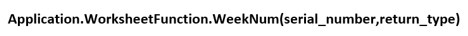The arguments in the above method have the same interpretation as explained in the WEEKNUM() Excel Formula section.

The following illustration explains how to use the above method.

The table below shows a list of dates.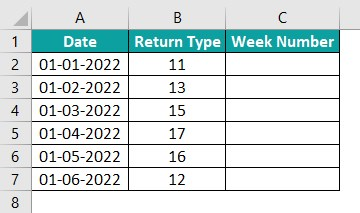Suppose the requirement is to determine the week number of each given date based on the specified return type or the day the corresponding week starts. And assume we need to display the result in column C.

Then, here is how to apply the WEEKNUM() in Excel VBA to achieve the required output.

Step 1: Press Alt + F11 to open the VBA Editor.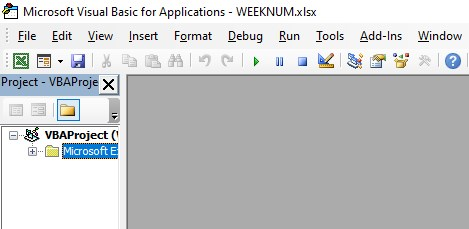Step 2: Pick the required VBAProject and select Insert à Module to open a new module window, Module1.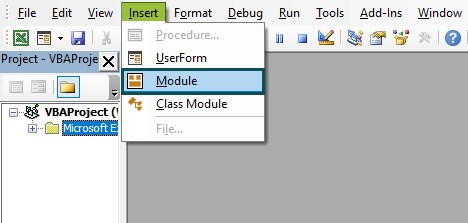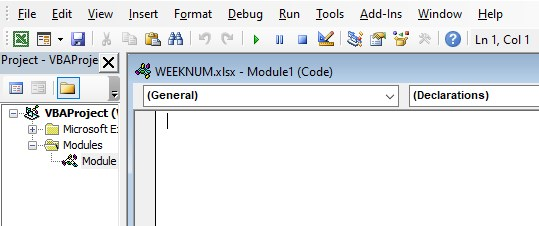Step 3: Enter the required code in the Module1 window to apply the WEEKNUM function.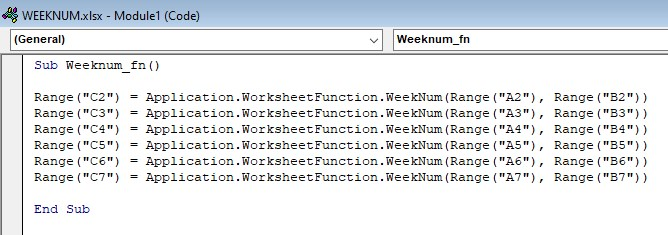Step 4: Click the Run Sub/UserForm icon in the top menu to run the code.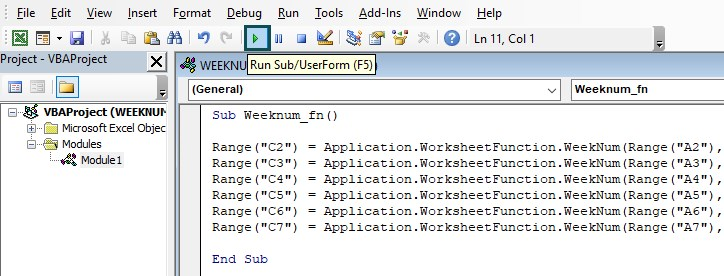And once the code gets executed, we will get the required week numbers in the target cells.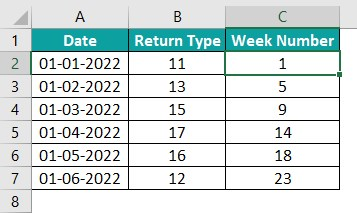The WEEKNUM method takes the date and return type values as the input. And, based on the day the week starts, the function returns the week number of the given date in each target cell.

2. What is the difference between the functions WEEKNUM and ISOWEEKNUM?

The difference between the functions WEEKNUM and ISOWEEKNUM is that the WEEKNUM() considers the week containing January 1st as week 1 of the year. So, in this case, the system will be System 1. And if the user wishes to follow System 2, the WEEKNUM() considers the week containing the year’s first Thursday as week 1 of the year. And thus, in this case, the WEEKNUM() will follow the ISO 8601-week numbering standard. But the return_type argument value table includes the value only for Monday for System 2, 21.

On the other hand, the ISOWEEKNUM() adheres to the ISO 8601 week numbering standard. Therefore, it considers only the week containing the year’s first Thursday as the year’s first week.

3. Why is the WEEKNUM function in Excel not working?

The WEEKNUM function in Excel is not working, perhaps due to the following reasons:

• We supplied a numeric but a date value out of the current date range as the first argument to the WEEKNUM().
• We supplied a non-numeric value as the first argument to the WEEKNUM().
• The provided return_type argument value is invalid.